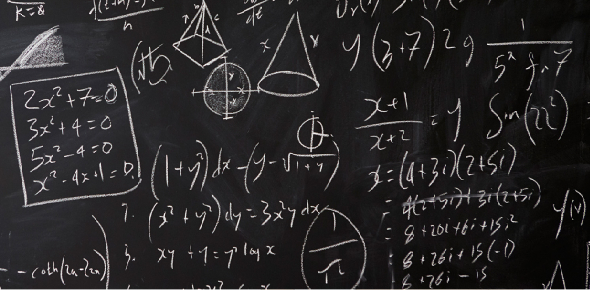# Slope-intercept Form

4 QuestionsSettingsPut the following in slope intercept form and find x and y intercept from the following equation

Related Topics
• 1.
Convert this equation to slope intercept form 5x + 3y =9
• A.

Y= 3/5x + 3

• B.

Y = -5/3x + 3

• C.

Y = 5/3x + 5

• 2.
Convert this equation to slope intercept form 6x -4y =16
• A.

Y= 6/4x -4

• B.

Y=6/4x + 4

• C.

Y=4x -4

• 3.
Find the x and y intercept from the following equation 3x -4y = 10
• A.

X = 3 y = 4

• B.

X = 10/3 y = 2

• C.

X = 10/3 y = -4

• 4.
Find the x and y intercept from the following equation -x + 2y =10
• A.

X =-10 y = 5

• B.

X = 5 y = -10

• C.

X = 2 y = 5